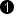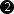## Operator ConvertPixelFormat

The operator ConvertPixelFormat tries to preserve as much information as possible, while arithmetic and bit width are adjusted to a desired output pixel format. Thus the operator allows the definition of the bit width and arithmetic at the output link.

The main principle of algorithm is twofold:

1. Change the arithmetic by clipping
• unsigned to signed: the MSB at the output is used as a sign bit
• signed to unsigned: clipping to positive values i.e. if a value is less than 0 it is set to zero
2. Change the bit width y keeping the relative brightness
• if the output bit width is increased, the value is left shifted to meet the desired bit width
• if the output bit width is decreased, the value is divided and rounded to meet the desired bit width

Thus the output value can be determined by:

Input Bit Width Output Bit Width Input Arithmetic Output Arithmetic Input Value Output Value Comment
Decimal Binary Decimal Binary
4 5 unsigned unsigned 10 1010 20 10100 value * 2
5 4 unsigned unsigned 15 1111 8 1000 value divided by 2 and rounded
5 4 unsigned unsigned 31 11111 15 1111 value clipped to maximum
4 4 unsigned signed 11 1011 6 0100 value divided by 2 and rounded
4 4 signed unsigned 4 0100 8 1000 value * 2
5 4 signed unsigned 10 01010 10 1010 value kept
5 4 signed unsigned -2 11110 0 0000 value clipped to 0
5 4 signed signed -7 11001 -4 1100 value divided by 2 and rounded

Table 23. Examples

For color links, each color component is processed individually.

### I/O Properties

Property Value
Operator Type O

Bit Width [1, 64][1, 64]Arithmetic {unsigned, signed} {unsigned, signed}
Parallelism any as I
Kernel Columns any as I
Kernel Rows any as I
Img Protocol {VALT_IMAGE2D, VALT_LINE1D, VALT_PIXEL0D} as I
Color Format any as I
Color Flavor any as I
Max. Img Width any as I
Max. Img Height any as I
 The range of the input bit width is [1, 64]. For signed inputs, the range is [2, 64]. For unsigned color inputs, the range is [3, 63] and for signed color, the range is [6, 63]. The range of the output bit width is [1, 64]. For signed outputs, the range is [2, 64]. For unsigned color outputs, the range is [3, 63] and for signed color, the range is [6, 63].

None

### Examples of Use

The use of operator ConvertPixelFormat is shown in the following examples: